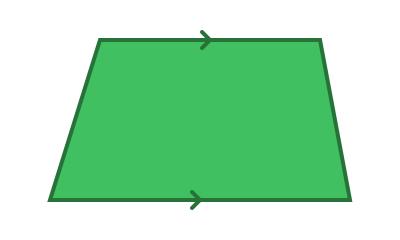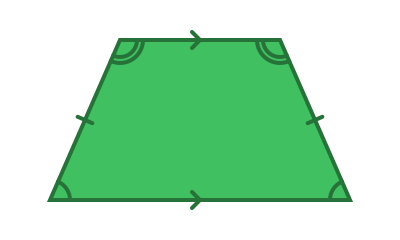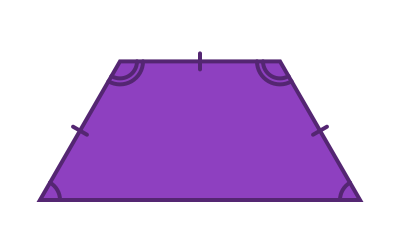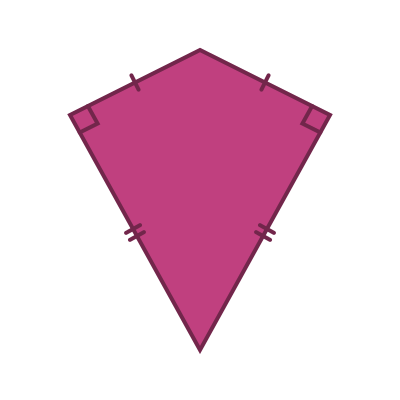Martin McBride
2020-08-14

Here are a few other, slightly less well known quadrilaterals.

## Types of trapezium

A trapezium is a quadrilateral that has at least two parallel sides.You can think of a trapezium as being a bit like a triangle with the top sliced off. Just like triangles, there are several different types of trapezium.

### Acute trapeziums

An acute trapezium is like a slice through an acute triangle.Notice that sides slope in opposite directions, and both angles at the base are acute.

### Obtuse trapeziums

An obtuse trapezium is like a slice through an obtuse triangle.Notice that one side slopes inwards, one slopes outwards. One angle at the base is acute, the other is obtuse.

### Right angled trapeziums

An right angled trapezium is like a slice through a right angled triangle.### Trapeziums with three equal sides

We have seen the isosceles trapezium in the quadrilaterals section. It is a trapezium where the two sides make the same angle to the base (but with opposite direction of slope). A you have probably guessed, it is a slice through and isosceles triangle.A special case of this trapezium is one where three of the sides are equal length:## Right kite

A kite is a quadrilateral that has two pairs of equal length sides that are adjacent to each other.

A kite always has two equal angles - the angles between a short and long side. A right kite is a special case where those angles are right angles.An interesting fact about right kites is they are cyclic - you can draw a circle that touches all four corners.